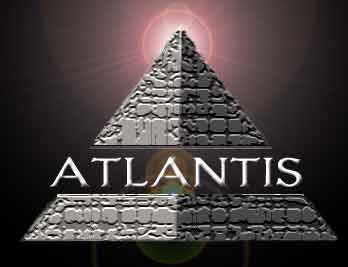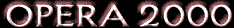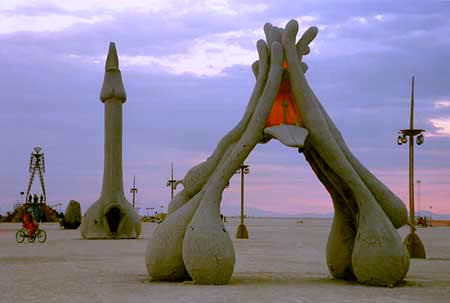# ~ ATLANTEAN LANGUAGE~

Our research and listening to the voices have led us to many interesting findings. One is that the Atlanteans originally used only seven consonants before coming to earth. However, either their voice adapted to this atmosphere or humans could not easily speak Atlantean with so little letters, so eventually three vowels were added.

When they spoke a word in Atlantean, each letter would be pronounced with a melodic voice. As a result, in ancient Atlantean, "Atlantis" was "Tlnts", difficult indeed! But eventually it became "Atilianoti-isa."

Two ways to translate English words into Atlantean

1) Rules for translating between English and Atlantean can be found below under "Instructions."

2) A short-cut is to use the handy

Pure-Loving-Atlantean-O-Matic Translator

*Special note to those Atlanteans amongst us*

Interested in translating your Atlantean into English? Check out

Instructions

1. Take a word (for example, "Daniel")
2. Translate each letter using the Atlantean pronunciation in the table below(for our example, "Tianiiili")
3. When there are two identical consecutive vowels, add a dash (for our example, "Tiani-i-ili")
4. If desired, a phonetic version of the word to be translated can be used instead. For example, "Filip" can be used instead of "Philippe", producing "Paili-ipa" instead of "Paxaili-ipapai."

English letter(s) = Atlantean letter(s) = Pronunciation = Mystic Name = Letter #

m,n = n = no = Iona = 1
s, z, (soft) c = s = sa = Netsa = 2
r,l = l = li = Linkan = 3
h, ch = xa = xat = Xat (pronounced "chat") = 4
e,y,i = i = ee = Isiti = 5
a = a = aah = Tipalit = 6
o, u, oo = o = ooh = Kipola = 7
k,g,c = k = ka = Xokna = 8
t, d, th = t = ti = Kiti = 9
p,b,v,f = p = pa = Pina = 10

English letter = Atlantean letter(s) = Pronunciation

j, (soft)g = ia = ia
w = oa = oa
q = koa = koa
x = ks = ksa
.

Apapai tilianosaliati-inokaia! (happy translating!)